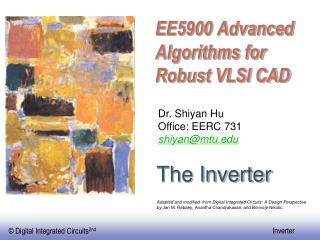Download Presentation# EE5900 Advanced Algorithms for Robust VLSI CAD - PowerPoint PPT PresentationDownload Presentation- - - - - - - - - - - - - - - - - - - - - - - - - - - E N D - - - - - - - - - - - - - - - - - - - - - - - - - - -
##### Presentation Transcript

1. EE5900 Advanced Algorithms for Robust VLSI CAD Dr. Shiyan Hu Office: EERC 731 shiyan@mtu.edu The Inverter Adapted and modified from Digital Integrated Circuits: A Design Perspective by Jan M. Rabaey, Anantha Chandrakasan, and Borivoje Nikolic.

2. Circuit Symbols

3. V DD S D V V in out D C L S The CMOS Inverter: A First Glance Vin=Vdd,Vout=0 Vin=0,Vout=Vdd

4. V V DD DD R p V out V out R n V V V 0 in DD in CMOS Inverter - First-Order DC Analysis

5. CMOS Inverter: Transient Response V V DD DD Delay=0.69RC R p V out V out C L C L R n V 0 V V in DD in (a) Low-to-high (b) High-to-low

6. NMOS In Inverter V DD S D V V in out D C L S • For NMOS • Vin=0, Vgsn=0<Vtn, Vdsn=Vout=Vdd, NMOS is in cut-off region, X1. • PMOS is on. Vout=Vdd. • Vin=Vdd, instantaneously, Vgsn=Vdd>Vtn,Vdsn=Vout=Vdd, Vgsn-Vtn=Vdd-Vtn<Vdd, NMOS is in saturation region, X2 • Instantaneously, Vgsp=0>Vtp. PMOS cut-off • NMOS is on so Vdsn->0. The operating point follows the arrow to the origin. Vout=0 at X3.

7. Propagation Delay

8. Rising delay and Falling delay Rising delay tr=time for the signal to change from 10% to 90% of Vdd Falling delay tf=time for the signal to change from 90% to 10% of Vdd Delay=time from input signal transition (50% Vdd) to output signal transition (50% Vdd).

9. Delay

10. Inverter falling-time

11. NMOS falling time V DD S D V V in out D C L S • For NMOS • Vin=0, Vgsn=0<Vt, Vdsn=Vout=Vdd, NMOS is in cut-off region, X1 • Vin=Vdd, instantaneously, Vgsn=Vdd>Vt,Vdsn=Vout=Vdd, Vgsn-Vtn=Vdd-Vtn<Vdd, NMOS is in saturation region, X2 • The operating point follows the arrow to the origin. So Vout=0 at X3.

12. NMOS falling time When Vin=Vdd, instantaneously, Vgsn=Vdd tf=tf1+tf2 tf1: time for CL to switch from 0.9Vdd to Vgsn-Vtn=Vdd-Vtn tf2: time for CL to switch from Vdd-Vtn to 0.1Vdd tf1 tf2

13. NMOS falling time For tf1: Integrate Vout from 0.9Vdd to Vdd-Vt For tf2, we have Vgsn=Vdd Vdsn=Vout

14. NMOS falling time tf=tf1+tf2 Assume Vt=0.2Vdd

15. Rising time Assume |Vtp|=0.2Vdd

16. Falling and Rising time Assume Vtn=-Vtp, then we can show that Thus, for equal rising and falling time, set That is, Wp=2Wn since up=un/2

17. Power Dissipation

18. Where Does Power Go in CMOS?

19. Vdd Vin Vout C L Dynamic Power Dissipation 2 Power = C * V * f L dd Not a function of transistor sizes Need to reduce C , V , and f to reduce power. L dd

20. Dynamic Power Dynamic power is due to charging/discharging load capacitor CL In charging, CL is loaded with a charge CL Vdd which requires the energy of QVdd= CL Vdd2, and all the energy will be dissipated when discharging is done. Total power = CL Vdd2 If this is performed with frequency f, clearly, total power = CL Vdd2 f

21. Dynamic Power- II • If the waveform is not periodic, denote by P the probability of switching for the signal • The dynamic power is the most important power source • It is quadratically dependant on Vdd • It is proportional to the number of switching. We can slow down the clock not on the timing critical path to save power. • It is independent of transistor size since it only depends on the load of the transistor.

22. Short Circuit Currents Happens when both transistors are on. If every switching is instantaneous, then no short circuits. Longer delay -> larger short circuit power

23. Short-Circuit Currents

24. Leakage Sub-threshold current one of most compelling issues in low-energy circuit design.

25. Subthreshold Leakage Component

26. Principles for Power Reduction • Prime choice: Reduce voltage • Recent years have seen an acceleration in supply voltage reduction • Design at very low voltages still open question (0.5V) • Reduce switching activity • Reduce physical capacitance

27. Impact ofTechnology Scaling

28. Goals of Technology Scaling • Make things cheaper: • Want to sell more functions (transistors) per chip for the same money • Build same products cheaper, sell the same part for less money • Price of a transistor has to be reduced • But also want to be faster, smaller, lower power

29. Scaling • Goals of scaling the dimensions by 30%: • Reduce gate delay by 30% • Double transistor density • Die size used to increase by 14% per generation • Technology generation spans 2-3 years

30. Technology Scaling • Devices scale to smaller dimensions with advancing technology. • A scaling factor S describes the ratio of dimension between the old technology and the new technology. In practice, S=1.2-1.5.

31. Technology Scaling - II • In practice, it is not feasible to scale voltage since different ICs in the system may have different Vdd. This may require extremely complex additional circuits. We can only allow very few different levels of Vdd. • In technology scaling, we often have fixed voltage scaling model. • W,L,tox scales down by 1/S • Vdd, Vt unchanged • Area scales down by 1/S2 • Cox scales up by S due to tox • Gate capacitance = CoxWL scales down by 1/S • scales up by S • Linear and saturation region current scales up by S • Current density scales up by S3 • P=Vdd*I, power density scales up by S3 • Power consumption is a major design issue

32. Summary Inverter Inverter delay Power Dynamic Leakage Short-circuit Technology scaling